Question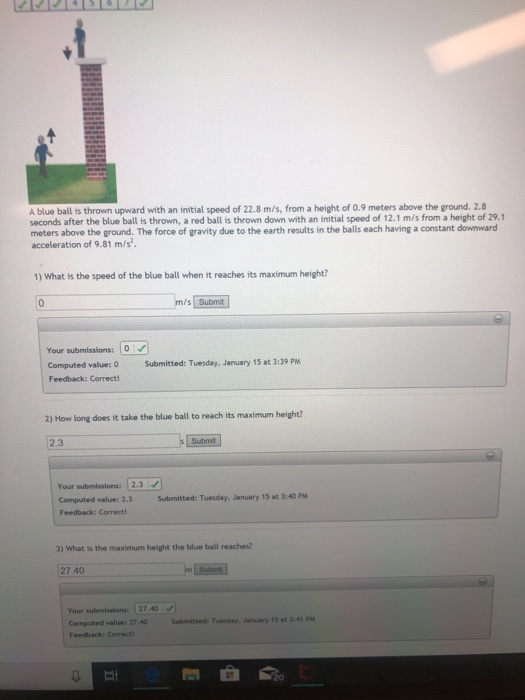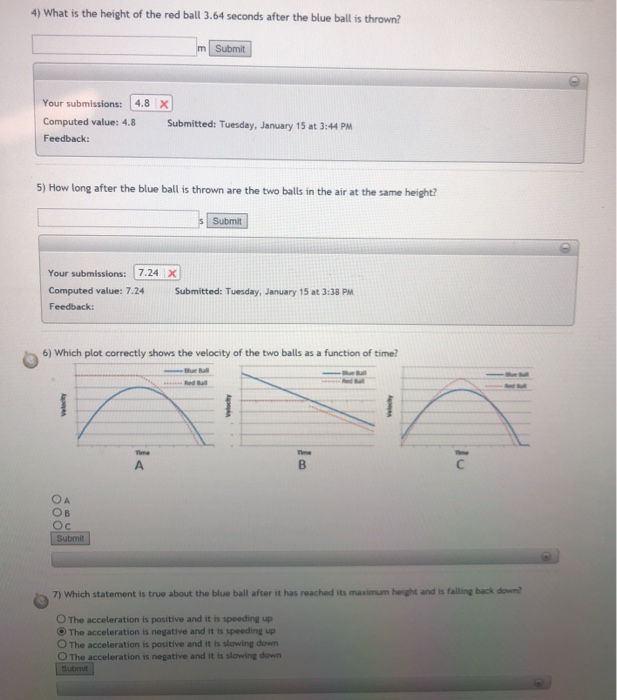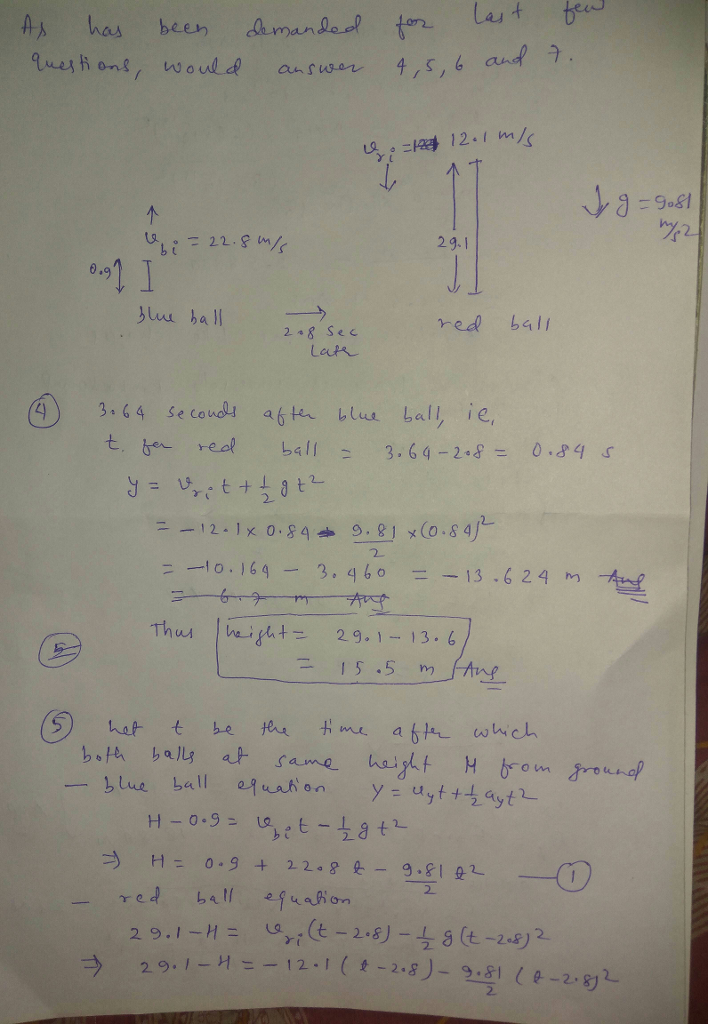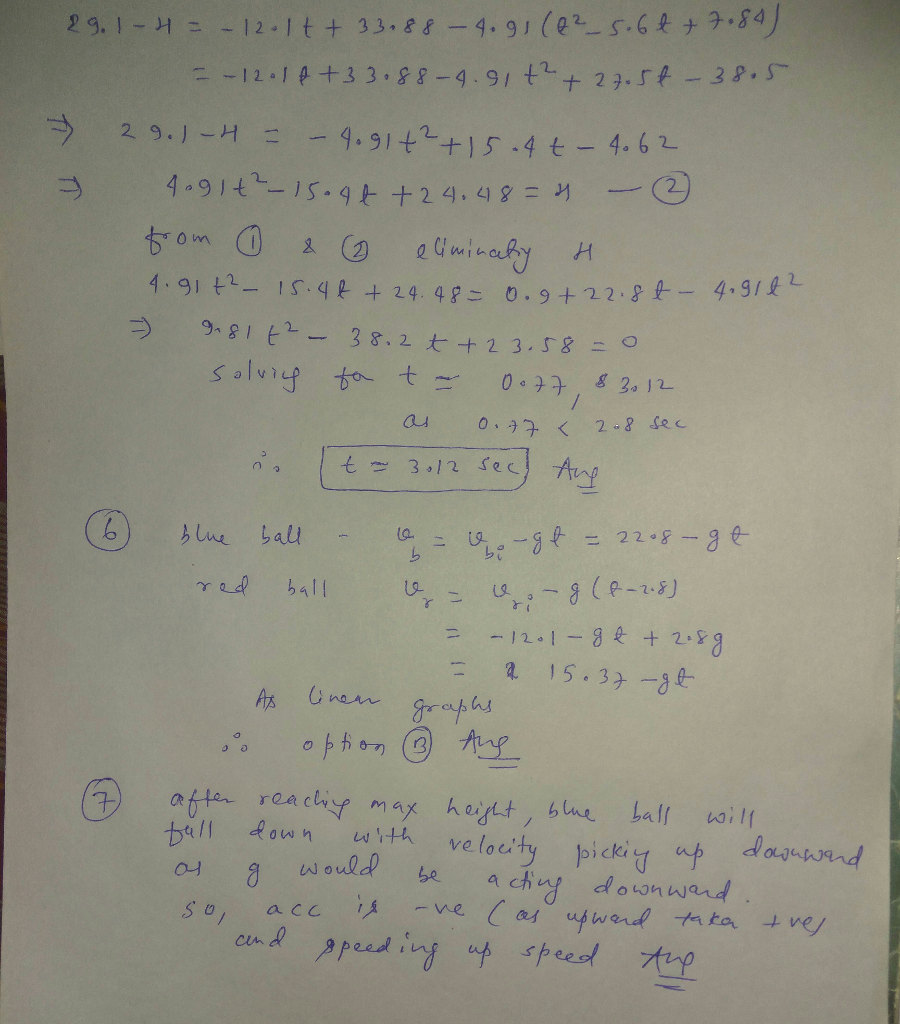#### Earn Coins

Coins can be redeemed for fabulous gifts.

Similar Homework Help Questions
• ### Two Thrown Balls 1 2 3 45678 A blue ball is thrown upward with an initial...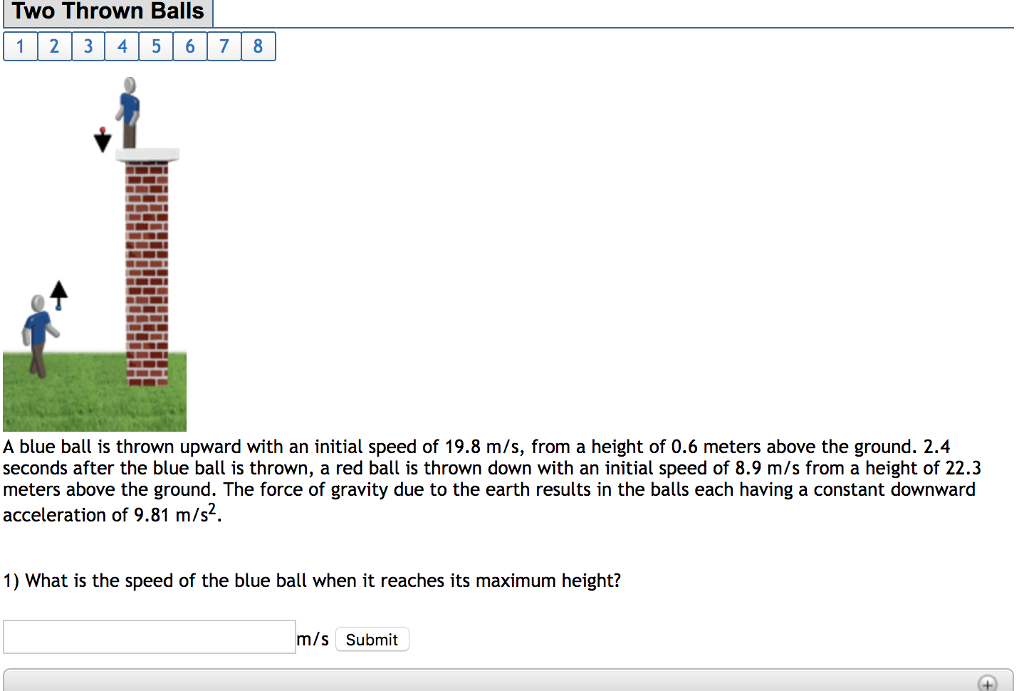Two Thrown Balls 1 2 3 45678 A blue ball is thrown upward with an initial speed of 19.8 m/s, from a height of 0.6 meters above the ground. 2.4 seconds after the blue ball is thrown, a red ball is thrown down with an initial speed of 8.9 m/s from a height of 22.3 meters above the ground. The force of gravity due to the earth results in the bals each having a constant downward acceleration of 9.81 m/s2....

• ### Two throw A blue ball is thrown upward with an initial speed of 22.2 m/s, from...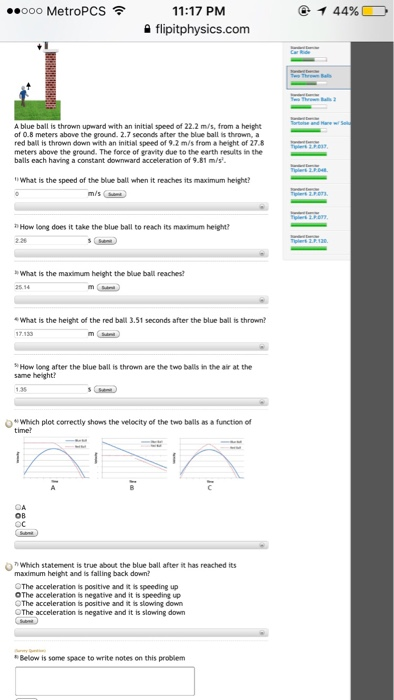Two throw A blue ball is thrown upward with an initial speed of 22.2 m/s, from a height of 0.8 meters above the ground. 2.7 seconds after the blue ball is thrown, a red ball is thrown down with an initial speed of 9.2 m/s from a height of 27.8 meters above the ground. The force of grandly due to the earth results in the balls each having a constant downward acceleration of 9.81 m/s^2. What is the speed of...

• ### A blue ball is thrown upward with an initial speed of 24.1 m/s, from a height...

A blue ball is thrown upward with an initial speed of 24.1 m/s, from a height of 0.8 meters above the ground. 2.9 seconds after the blue ball is thrown, a red ball is thrown down with an initial speed of 10.4 m/s from a height of 31.4 meters above the ground. The force of gravity due to the earth results in the balls each having a constant downward acceleration of 9.81 m/s2. What is the height of the red...

• ### A blue ball is thrown upward with an initial speed of 19.8 m/s, from a height of 0.5 meters above the ground

A blue ball is thrown upward with an initial speed of 19.8 m/s, from a height of 0.5 meters above the ground. 2.4 seconds after the blue ball is thrown, a red ball is thrown down with an initial speed of 7.9 m/s from a height of 22.5 meters above the ground. The force of gravity due to the earth results in the balls each having a constant downward acceleration of 9.81 m/s2. What is the maximum height the blue...

• ### A blue ball is thrown upward with an initial speed of 23.9 m/s, from a height...

A blue ball is thrown upward with an initial speed of 23.9 m/s, from a height of 0.5 meters above the ground. 2.9 seconds after the blue ball is thrown, a red ball is thrown down with an initial speed of 7.5 m/s from a height of 31.5 meters above the ground. The force of gravity due to the earth results in the balls each having a constant downward acceleration of 9.81 m/s^2. How long after the blue ball is...

• ### A blue ball is thrown upward with an initial speed of 20 m/s, from a height...

A blue ball is thrown upward with an initial speed of 20 m/s, from a height of 0.8 meters above the ground. 2.4 seconds after the blue ball is thrown, a red ball is thrown down with an initial speed of 5.3 m/s from a height of 22.4 meters above the ground. The force of gravity due to the earth results in the balls each having a constant downward acceleration of 9.81 m/s2. 1) How long after the blue ball...

• ### Two cars start from rest at a red stop light. When the light turns green, both...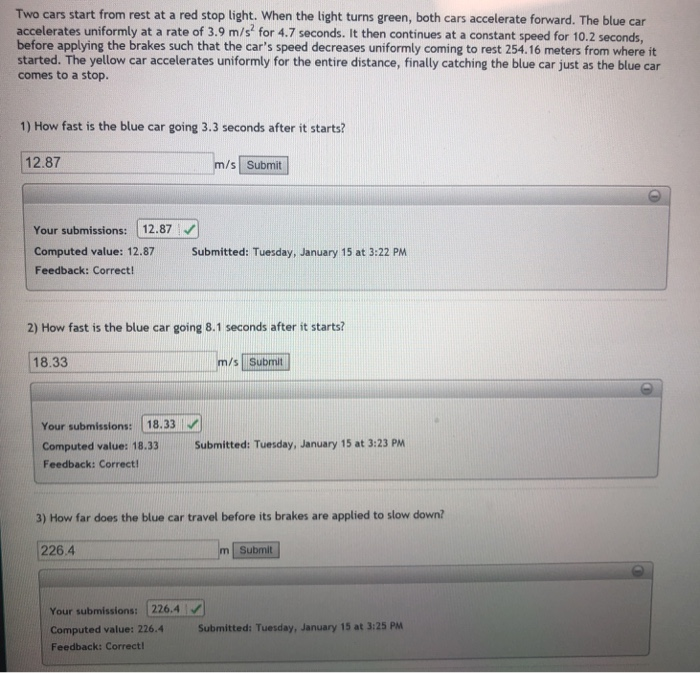Two cars start from rest at a red stop light. When the light turns green, both cars accelerate forward. The blue car accelerates uniformly at a rate of 3.9 m/s2 for 4.7 seconds. It then continues at a constant speed for 10.2 seconds, before applying the brakes such that the car's speed decreases uniformly coming to rest 254.16 meters from where it started. The yellow car accelerates uniformly for the entire distance, finally catching the blue car just as the...

• ### A red ball is thrown down with an initial speed of 1.3 m/s from a height...

A red ball is thrown down with an initial speed of 1.3 m/s from a height of 26 meters above the ground. Then, 0.4 seconds after the red ball is thrown, a blue ball is thrown upward with an initial speed of 23.9 m/s, from a height of 1 meters above the ground. The force of gravity due to the earth results in the balls each having a constant downward acceleration of 9.81 m/s2. What is the speed of the...

• ### A red ball is thrown down with an initial speed of 1.2 m/s from a height...

A red ball is thrown down with an initial speed of 1.2 m/s from a height of 25 meters above the ground. Then, 0.6 seconds after the red ball is thrown, a blue ball is thrown upward with an initial speed of 23.8 m/s, from a height of 0.8 meters above the ground. The force of gravity due to the earth results in the balls each having a constant downward acceleration of 9.81 m/s^2. 1)What is the speed of the...

• ### A red ball is thrown down with an initial speed of 1.2 m/s from a height...

A red ball is thrown down with an initial speed of 1.2 m/s from a height of 26 meters above the ground. Then, 0.4 seconds after the red ball is thrown, a blue ball is thrown upward with an initial speed of 23.8 m/s, from a height of 1 meters above the ground. The force of gravity due to the earth results in the balls each having a constant downward acceleration of 9.81 m/s2. 1) What is the speed of...